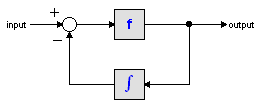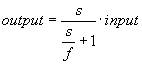﻿ 20-sim webhelp > Library > Iconic Diagrams > Mechanical > Rotation > Actuators > positionactuator-relative

positionactuator-relative

Library

Iconic Diagrams\Mechanical\Rotation\Actuators

Use

Domains: Continuous. Size: 1-D. Kind: Iconic Diagrams (Rotation), Block Diagrams.

Description

This model represents an ideal actuator. An angle input signal is differentiated by a state variable filter to an angular velocity difference between its two terminals:

p_high.omega = p_low.omega + dphi/dt;

p_low.T = p_high.T = indifferent;

Differentiation is performed by a state variable filter:The S-domain function of this filter is equal to:where f is the cut-off frequency. For very high values of f, the output becomes the pure derivative of the input. High values of f, however, increase simulations times. A good trade-off is a starting value of 1e5.

Interface

 Ports Description p_high, p_low Both terminals of the Rotation port p. Causality fixed angular velocity out Input phi Angle [rad]. Parameters f omega_initial Cut-off frequency of the differentiation [Hz]. Initial angular velocity output of the differentiation [rad/s].